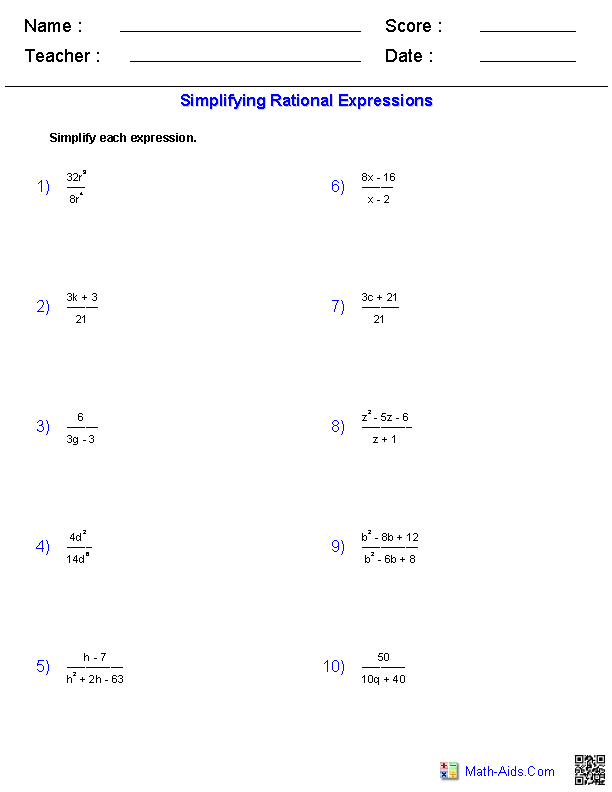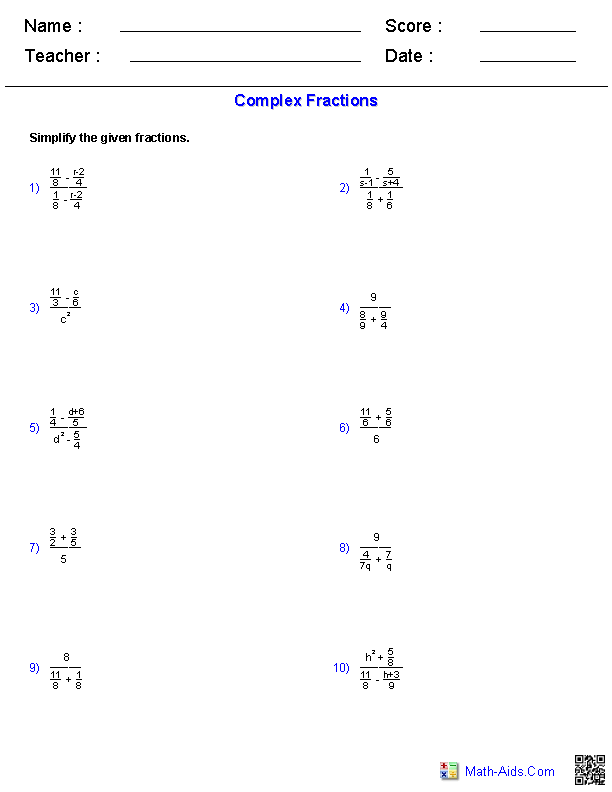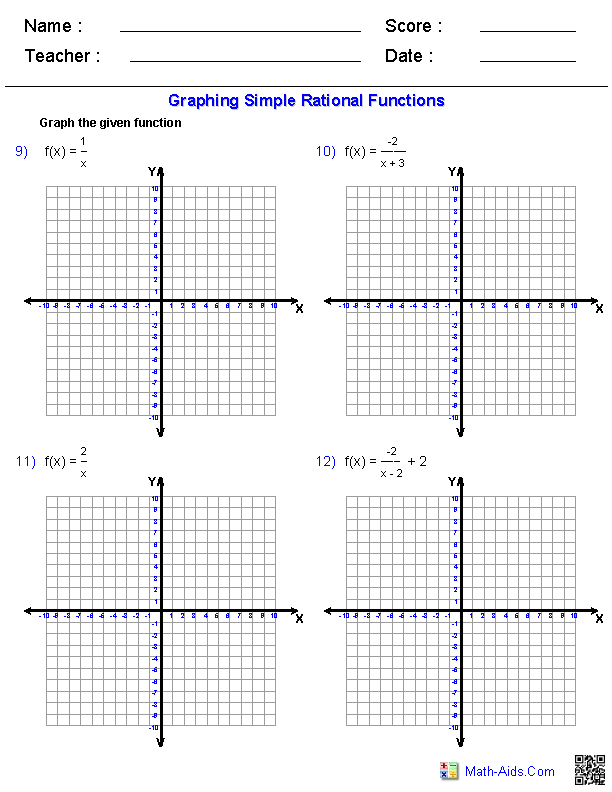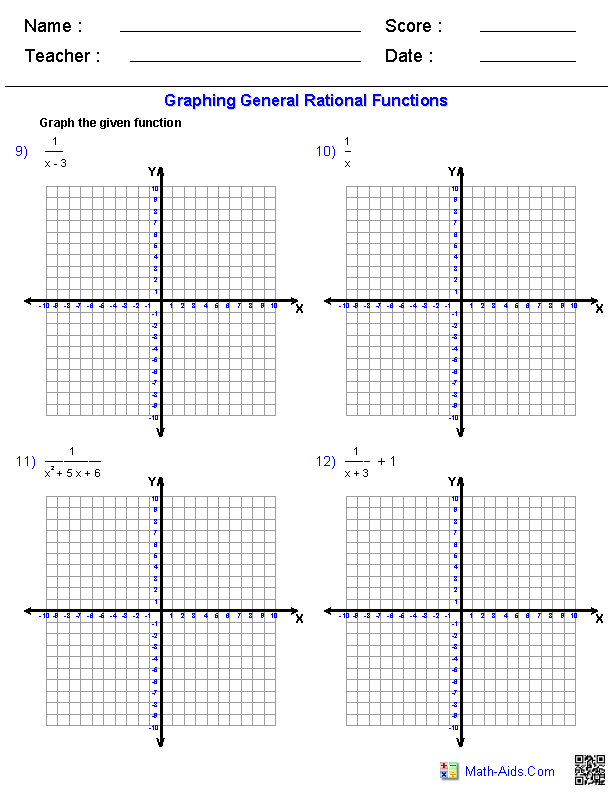#Algebra 2 Worksheets

## Rational Expressions Worksheets

Here is a graphic preview for all of the Rational Expressions Worksheets. You can select different variables to customize these Rational Expressions Worksheets for your needs. The Rational Expressions Worksheets are randomly created and will never repeat so you have an endless supply of quality Rational Expressions Worksheets to use in the classroom or at home. Our Rational Expressions Worksheets are free to download, easy to use, and very flexible.

These Rational Expressions Worksheets are a good resource for students in the 8th Grade through the 12th Grade.

Click here for a Detailed Description of all the Rational Expressions Worksheets.

## Quick Link for All Rational Expressions Worksheets

Click the image to be taken to that Rational Expressions Worksheets.

##### SimplifyingRational Expressions Worksheets##### Adding and SubtractingRational Expressions Worksheets##### Multiplying and DividingRational Expressions Worksheets##### Solving Rational EquationsRational Expressions Worksheets##### Complex FractionsRational Expressions Worksheets##### Graphing SimpleRational FunctionsRational Expressions Worksheets##### Graphing GeneralRational FunctionsRational Expressions WorksheetsRecommended Videos

## Description For All Rational Expressions Worksheets

Simplifying Rational Expressions Worksheets
This Rational Expressions worksheet will produce problems for simplifying rational expressions. You may select the types of denominators you want in each expression. This Rational Expression worksheet will produce ten problems per page. This Rational Expressions worksheet is a good resource for students in the 9th Grade, 10th Grade, 11th Grade, and 12th Grade.

Adding and Subtracting Rational Expressions Worksheets
This Rational Expressions worksheet will produce problems for adding and subtracting rational expressions. You may select the types of denominators you want in each expression. This Rational Expression worksheet will produce ten problems per page. This Rational Expressions worksheet is a good resource for students in the 9th Grade, 10th Grade, 11th Grade, and 12th Grade.

Multiplying and Dividing Rational Expressions Worksheets
This Rational Expressions worksheet will produce problems for multiplying and dividing rational expressions. You may select the types of numerators and denominators you want in each expression. This Rational Expression worksheet will produce ten problems per page. This Rational Expressions worksheet is a good resource for students in the 9th Grade, 10th Grade, 11th Grade, and 12th Grade.

Solving Rational Equations Worksheets
This Rational Expressions worksheet will produce problems for solving rational expressions. You may select the types of numerators and denominators you want in each expression. This Rational Expression worksheet will produce ten problems per page. This Rational Expressions worksheet is a good resource for students in the 9th Grade, 10th Grade, 11th Grade, and 12th Grade.

Complex Fractions Worksheets
This Rational Expressions worksheet will produce problems for Complex Fractions. You may select the difficulty of the problems. This Rational Expression worksheet will produce ten problems per page. This Rational Expressions worksheet is a good resource for students in the 9th Grade, 10th Grade, 11th Grade, and 12th Grade.

Graphing Simple Rational Functions Worksheets
This Rational Expressions worksheet will produce problems for for graphing simple rational functions. You may select the types of rational functions to graph. This Rational Expression worksheet will produce twelve problems across two pages. This Rational Expressions worksheet is a good resource for students in the 9th Grade, 10th Grade, 11th Grade, and 12th Grade.

Graphing General Rational Functions Worksheets
This Rational Expressions worksheet will produce problems for for graphing general rational functions. You may select the types of rational functions to graph. This Rational Expression worksheet will produce twelve problems across two pages. This Rational Expressions worksheet is a good resource for students in the 9th Grade, 10th Grade, 11th Grade, and 12th Grade.